# Team:WFLA YK PAO/Model

WFLA_YK_PAO

MODEL
In order to analyze the relationship between the enzyme activity and the reaction time, we timely measured the absorbances at a wavelength of 240 nm (A240), which is the specific absorption of MHET.
MHETase Reactions were performed in triplicate at 30°C. Reaction mixtures contain 50 nM recombinant GST-MHETase, 5 mM MHET in 90 mM NaCl, 10% (v/v) DMSO, 45 mM sodium phosphate, pH 7.5. Reactions were terminated by adding an equal volume of methanol. The reactions using inactive GST-MHETase (obtained by boiling for 10 min) were used as blank controls.
The initial data is given below:
Table 1. A240 and the enzyme activity of the assay groups against reaction time *Enzyme Activity = (A240blank - A240assay)/A240blank *100%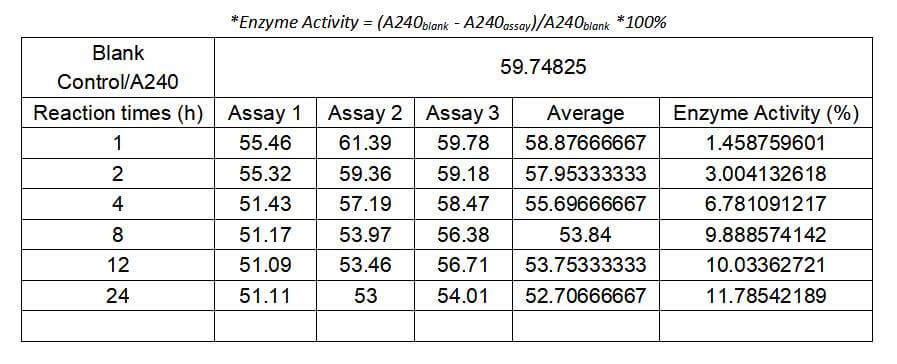According to the scatter plots (Fig. 1), we chose the cubic polynomial equation to adapt our data and model results are shown in figure 2.
The cubic polynomial equation: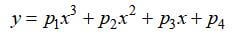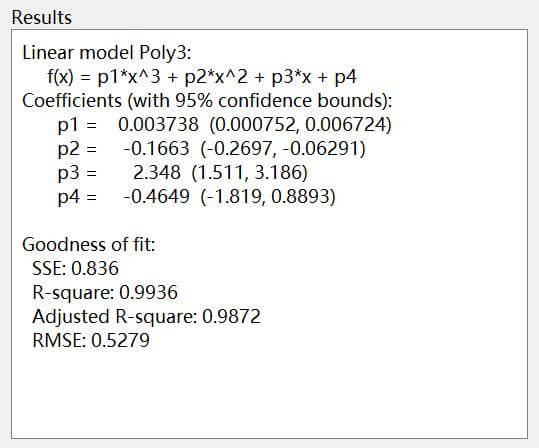Figure 1. The model result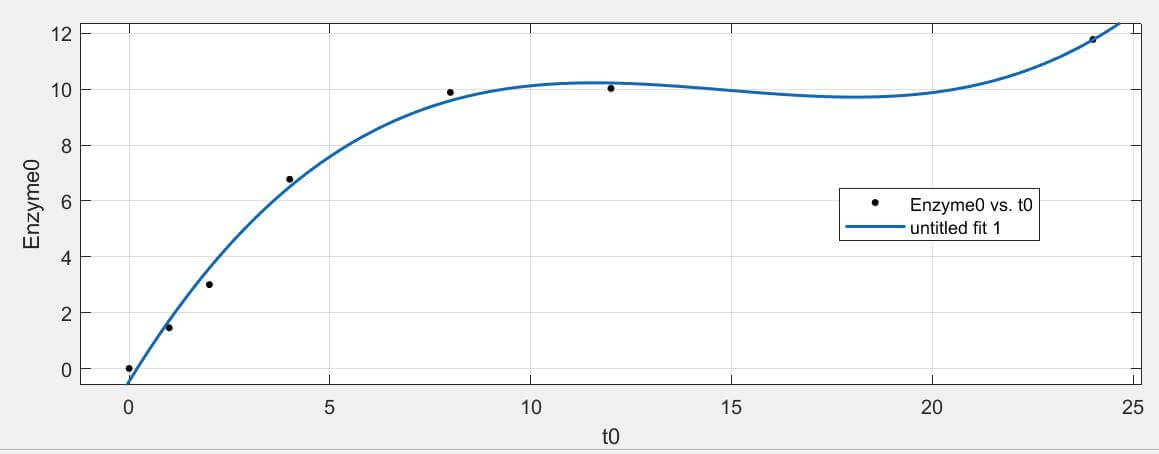Figure 2. The fitting curve
According to the fitting curve (Fig. 2), we can clearly see that there is an increasing trend of the enzyme activity against hours and the model fits well with the fitting degree, 0.9936 (Fig. 1). This model could be used to predict the most enzyme (MHETase) activity, namely, the most degradation percents of MHETase about MHET.
What is worth mentioning is that we also noticed the increasing rate which also changes as the reaction time goes by. In order to further explore the relationship between the reaction rate and the reaction time of the enzyme (MHETase), we further calculate the derivative formula of the model equation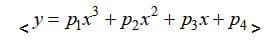and its curve (Fig. 3)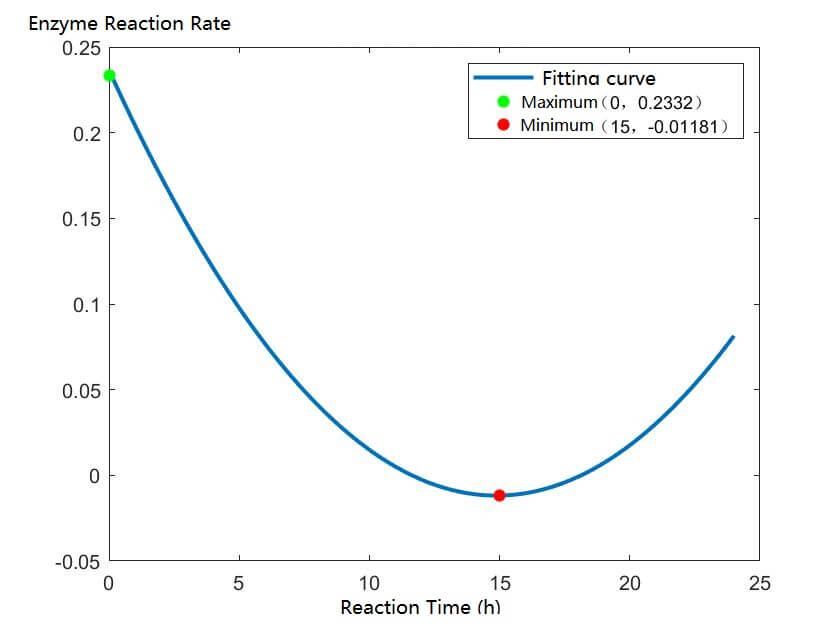Figure 3. The fitting curve of the relationship between the enzyme reaction rate and the reaction time of MHETase
In figure 3, it indicates that the enzyme reaches its greatest reaction rate at the very beginning and it usually takes 15 hours to reach the reaction balance (the reaction rate reaches 0 around). These values could be used as a reference when we further conduct the enzyme activity tests of IsPETase so as to determine the optimal duration of taking our product in the future.